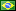###Author Topic: Avoid using DIV or IDIV when dividing by a constant  (Read 162 times)

####fredericopissarra

• Full Member
•• Posts: 308
• Country:##### Avoid using DIV or IDIV when dividing by a constant
« on: April 28, 2023, 01:52:29 PM »
Avoid using DIV or IDIV when dividing by a constant

The integer division instructions are, in Intel x86 processors, very slow. In old processors like 386/486 the latency was abour 100 clock cycles, in the old Pentium, 40, in "modern" Haswell microarchitecture it is 30 and in newest processors (10th generation), 17. Compare this to the multiplication instruction (MUL, IMUL), which takes 3~4 cycles only.

Is well known that if we want to divide two values (n/k), then this is the same as n*(1/k). If k is a constant, 1/k is a constant, but the problem is that we are dealing with integer division and for any k>1, 1/k will be always 0. To avoid this we can scale 1/k up, let's say multiplying by 2³² and getting 2³²/k. After the multiplication all we have to do is divide de result by 2³² to get the quotient.

Let's say we want to divide n (an unsigned integer) by 10, round(2³²/10) is 429496730. So we can do:
Code: [Select]
`; Entry: EDI=n; Exit: EAX=n/10div10:  mov  eax,edi  imul rax,rax,429496730  shr  rax,32  ret`This is equivalent to:
Code: [Select]
`; Entry: EDI=n; Exit: EAX=n/10div10:  mov  ecx,10  xor  edx,edx   ; this is needed, since EDX:EAX will be divided by ECX.  mov  eax,edi  div  ecx  ret`
The difference, of course, is that div will waste 17 cycles in modern processors (or 30, 40 or 100 in older ones), but imul will only waste 4.

If you take a look in code created by good compilers like GCC or CLANG for this, in C:
Code: [Select]
`unsigned int div10( unsigned int n ) { return n / 10U; }`You'll see a different constant:
Code: [Select]
`; Code createad by using -O2 option:div10:  mov     eax, edi  mov     edx, 3435973837  imul    rax, rdx  shr     rax, 35  ret`Why a different constant and why shifting 35 bits (not 32?). Because these compilers tries to minimize any possible erro using 32 bits of "significant" bit from the constant 1/k.

1/10, in binary, is 0b0.000110011001100... Then the compiler do:
Code: [Select]
`       bits to shift back added to 32.       |       |                                 this bit is 1, rouding        |                                 the significant bits up.       |                                 |      |-||----- 32 signifitive bits ----||  0b0.000110011001100110011001100110011001100...`That's why 3435973837 (or 0xCCCCCCCD) was used and 35 bits (32 + the the first 0's).

But, what if n is signed? like in:
Code: [Select]
`int idiv10(int x) { return x / 10; }`
The rules are the same, but we must reserve 1 bit for signal. Instead of scaling to 2³², we scale to 2³¹ and keep track of the signal of n to add 1, if negative (remember: in two's complement -n = ~n + 1), so the original routine is almost the same:
Code: [Select]
`; Entry: EDI=n; Exit: EAX=n/10idiv10:  mov  eax,edi  shr  edi,1              ; put the sign bit of EDI into bit 0.  imul rax,rax,214748365  ; 2³¹/10  shr  rax,32  add  eax,edi            ; add 1 if negative.  ret`In the case of GCC/CLANG they will, again, try to minimize possible errors, but still a 32 bits value, this time with the msb zeroed:
Code: [Select]
`idiv10:  movsx   rax, edi  sar     edi, 31  imul    rax, rax, 1717986919 ; = 0x66666667 (should be 0x66666666).  sar     rax, 34  sub     eax, edi  ret`Why 66666667, because it is the same value as before, but with the msb zeroed, hence 34 bits shift at the end, not 35:
Code: [Select]
`       bits to shift back added to 32.       |       |                                 this bit is 0, NOT rouding       |                                 the significant bits up is       |                                 what GCC should do!      +|                                 |      || |----- 32 signifitive bits ----||  0b0.00_0110011001100110011001100110011001100...         |         msb=0`
Again, even if we have 2 shifts and a subtraction, those instructions tend to be paralelized. The idiv10 routine takes, roughly 6 cycles to execute, using idiv instruction it would be, at least, 17 cycles (or more).